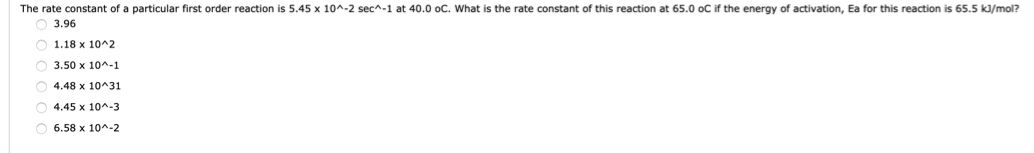# The rate constant of a particular first order reaction is 5.45 x 10^-2 secA-1 at 40.0 oC. What is the rate constant of this reaction at 65.0 oC if the energy of activation, Ea for this reaction is 65.5 kJ/mol? 3.96 1.18 x 10^2 3.50 x 10^-1 4.48 x 10^31 4.45 x 10^-3 6.58 x 10^-2